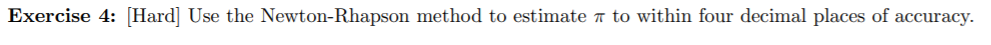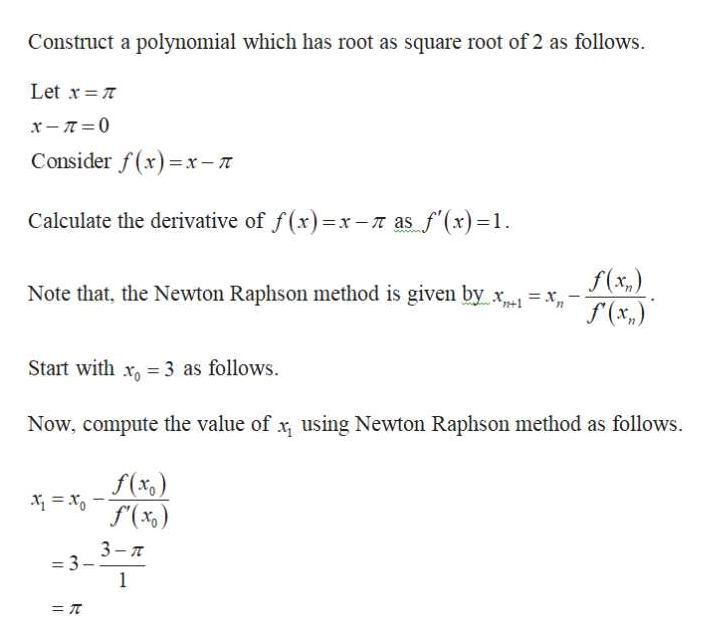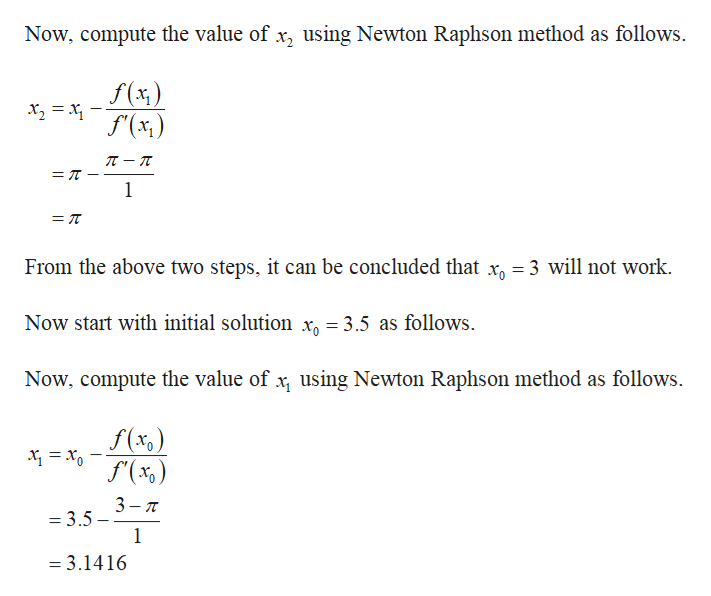# Exercise 4: [Hard] Use the Newton-Rhapson method to estimater to within four decimal places of accuracy.

Question
1 viewshelp_outlineImage TranscriptioncloseExercise 4: [Hard] Use the Newton-Rhapson method to estimater to within four decimal places of accuracy. fullscreen
check_circle

Step 1help_outlineImage TranscriptioncloseConstruct a polynomial which has root as square root of 2 as follows. Let x T x- 0 Consider f(x)x-T Calculate the derivative of f(x)=x-r as f(x)=1 wwww f(x,) (x,) Note that, the Newton Raphson method is given by Start with x 3 as follows Now, compute the value of x using Newton Raphson method as follows. f(xc 3-7 -3 1 =T fullscreen
Step 2help_outlineImage TranscriptioncloseNow, compute the value of x, using Newton Raphson method as follows f(x) f'(x,) л — п 1 From the above two steps, it can be concluded that x3 will not work. xg 3.5 as follows Now start with initial solution Now, compute the value of x using Newton Raphson method as follows f(x,) f(%) 3 = 3.5 1 = 3.1416 fullscreen

### Want to see the full answer?

See Solution

#### Want to see this answer and more?

Solutions are written by subject experts who are available 24/7. Questions are typically answered within 1 hour.*

See Solution
*Response times may vary by subject and question.
Tagged in

### Math